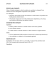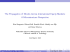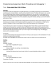# CSE 101 Homework 3 - UCSD VLSI CAD Laboratory

## Transcription

CSE 101 Homework 3 - UCSD VLSI CAD Laboratory
```CSE 101 Homework 3
February 5, 2015
CSE 101: Design and Analysis of Algorithms
Winter 2015
Homework 3
Due: February 12, 2015
1. Given an array of non-negative integers, you are initially positioned at the first index of the array
(we will call this index 0). Each element in the array represents your maximum jump length at that
position. Your goal is to reach the last index in the minimum number of jumps.
For example:
Suppose you are given array A = [2, 3, 1, 1, 4]. The minimum number of jumps to reach the last index
is 2. (Starting with index 0, move to index 1 with the first jump. Move 3 indices with the second jump
and reach index 4.)
Give a linear time algorithm to find the minimum number of jumps required to reach the last index.
0
2. A feedback edge set of an undirected graph G = (V, E) is a subset of edges E ⊂ E that intersects
0
every cycle of the graph. Thus, removing the edges E will render the graph acyclic.
Give an efficient algorithm for the following problem:
Input: Undirected graph G = (V, E) with positive edge weights w
Pe .
0
Output: A feedback edge set E ⊂ E of minimum total weight
we .
e∈E 0
3. (DPV textbook, Problem 5.20.) Give a linear-time algorithm that takes as input a tree and determines
whether it has a perfect matching: a set of edges that touches each node once.
4. (DPV textbook, Problem 5.32.) A server has n customers waiting to be served. The service time
required by each customer is known in advance: it is ti minutes for customer i. So if, for example, the
i
P
customers are served in order of increasing i, then the ith customer has to wait
tj minutes.
j=1
We wish to minimize the total waiting time
T =
n
P
(time spent waiting by customer i).
i=1
Give an efficient algorithm for computing the optimal order in which to process the customers.
5. (CLRS textbook, Problem 22.1-4.) Suppose that a weighted, directed graph G = (V, E) has a negativeweight cycle. Give an efficient algorithm to list the vertices of one such cycle. Prove that your algorithm
is correct.
6. Suppose you are only interested in finding shortest paths in directed acyclic graphs. Modify the
Bellman-Ford algorithm so that it finds the shortest paths from the starting vertex s ∈ V to all other
vertices in one iteration.
1
```

### CSE 101 Homework 4 - UCSD VLSI CAD Laboratory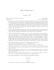### Engineering 9 – Statics Sample Test for Exam #2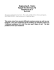### THE SCOOP BEHIND### CSE 255, Winter 2015: Homework 5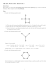### JUMP FOR DIABETES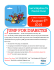### Document 6512841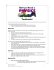### Registration Process for Lyryx I. Students without an account on Lyryx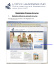### Homework 1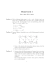### AccuPower sTAnDAlone soFTwAre FeATures MInIMuM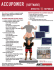### 1, 2, 3 Count and Move with Me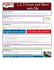### BITS WILP MSSS 2013-15 (S2-13_SSZG519) EC1 Quiz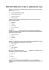### Fundamental Algorithms and Data Structures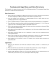### Lab 7 - ocal.info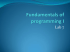### our sales catalogue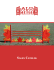### Model Specification and Risk Premia: Evidence from Futures Options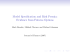### Invitation In English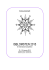### MCS 401 Exam 2 1 April 2015 Name: â¢ Do not start until instructed to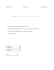### Part 1: - Dr. Aiman Hanna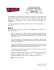### Lecture 5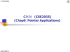### Decrease and Conquer